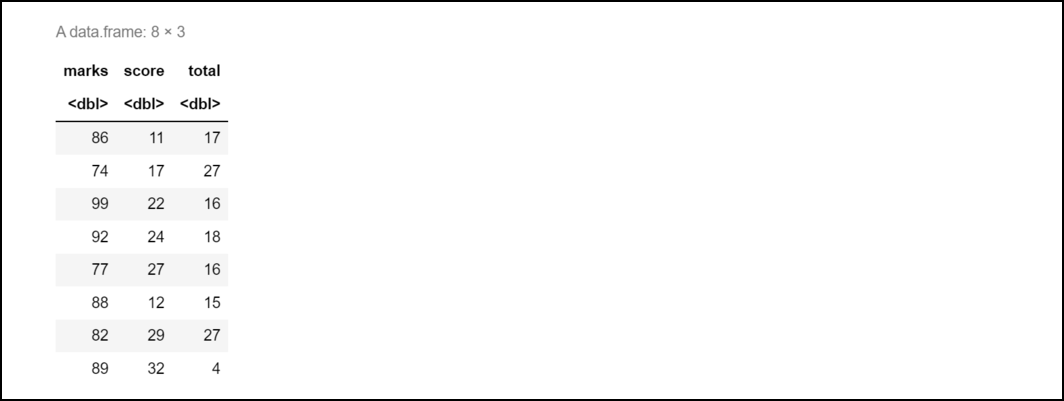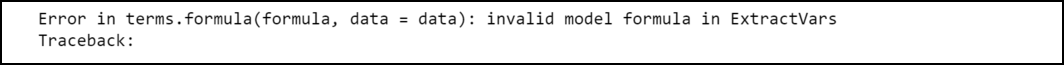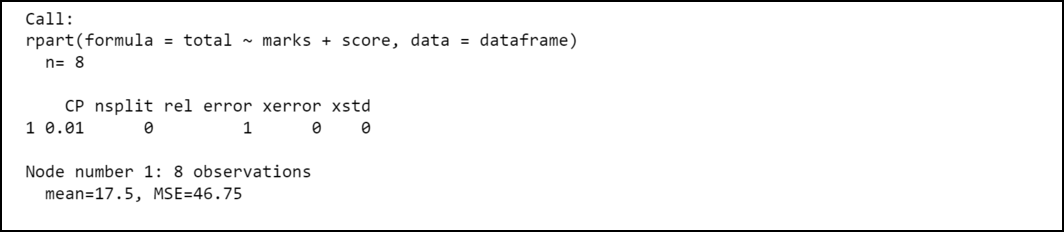GFG App
Open AppBrowser
Continue

# How to Fix in R: invalid model formula in ExtractVars

In this article, we will discuss how we can fix the “invalid model formula in ExtractVars” error in the R programming language.

#### The error that one may face in R is:

```Error in terms.formula(formula, data = data) :
invalid model formula in ExtractVars```

The R compiler produces such an error we try to fit a decision tree and use at least one variable incorrectly in the formula.

## When this error might occur in R

Let’s firstly create a data frame:

## R

 `# Make a data frame ` `dataframe <- ``data.frame``(marks=``c``(86, 74, 99, 92, 77, 88, 82, 89), ` `                 ``score=``c``(11, 17, 22, 24, 27, 12, 29, 32), ` `                 ``total=``c``(17, 27, 16, 18, 16, 15, 27, 4)) ` `dataframe`

Output:Here, we need to use the rpart function from the rpart package to fit a decision tree model and further evaluate it.

Syntax to install the rpart package in the R console:

install.package(‘rpart’)

Suppose that we want to use the rpart() function to fit a decision tree model in the data and then the R compiler produces the error of “invalid model formula in ExtractVars” because we have given quotations at the ends of the predictor variables.

## R

 `# Importing the library ` `library``(rpart) ` ` `  `# Make a data frame ` `dataframe <- ``data.frame``(marks=``c``(86, 74, 99, 92, 77, 88, 82, 89), ` `                 ``score=``c``(11, 17, 22, 24, 27, 12, 29, 32), ` `                 ``total=``c``(17, 27, 16, 18, 16, 15, 27, 4)) ` ` `  `# Try to fit decision tree model to data ` `model <- ``rpart``(total ~ ``"marks"` `+ ``"score"``, data = dataframe)`

Output:Output

## How to Fix the Error:

We can fix this error easily by simply removing quotations from the predictor variables and writing the formula as given in the code below:

## R

 `# Importing the library ` `library``(rpart) ` ` `  `# Make a data frame ` `dataframe <- ``data.frame``(marks=``c``(86, 74, 99, 92, 77, 88, 82, 89), ` `                 ``score=``c``(11, 17, 22, 24, 27, 12, 29, 32), ` `                 ``total=``c``(17, 27, 16, 18, 16, 15, 27, 4)) ` ` `  `# Try to fit decision tree model to data ` `model <- ``rpart``(total ~ marks + score, data = dataframe) ` ` `  `# Print the summary of the model ` `summary``(model)`

Output:My Personal Notes arrow_drop_up